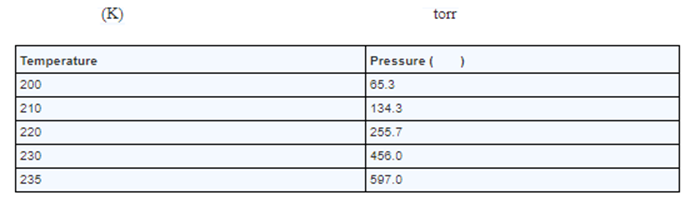Problem: The vapor pressure of ammonia at several different temperatures is shown below.a. Use the date to determine the heat of vaporization of ammoniab. Determine the normal boiling point of ammonia.

FREE Expert Solution
90% (243 ratings)
Problem Details

The vapor pressure of ammonia at several different temperatures is shown below.

a. Use the date to determine the heat of vaporization of ammonia

b. Determine the normal boiling point of ammonia.What scientific concept do you need to know in order to solve this problem?

Our tutors have indicated that to solve this problem you will need to apply the Clausius-Clapeyron Equation concept. You can view video lessons to learn Clausius-Clapeyron Equation Or if you need more Clausius-Clapeyron Equation practice, you can also practice Clausius-Clapeyron Equation practice problems .

What is the difficulty of this problem?

Our tutors rated the difficulty of The vapor pressure of ammonia at several different temperatu... as high difficulty.

How long does this problem take to solve?

Our expert Chemistry tutor, Dasha took 10 minutes to solve this problem. You can follow their steps in the video explanation above.

What professor is this problem relevant for?

Based on our data, we think this problem is relevant for Professor Pan's class at UA.